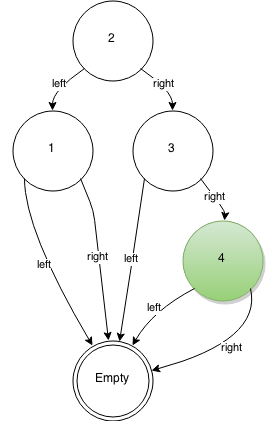# Exploring the .NET CoreFX Part 13: ImmutableList is an AVL Tree

This is part 13 of my Exploring the .NET CoreFX Series.

Most implementations of `IList`, including `System.Collections.Generic.List`, are dynamic arrays. `System.Collections.Immutable.ImmutableList` is different – it is an AVL tree. This results in significantly different performance characteristics:

`List` `ImmutableList`
Indexing O(1) O(log n)
Append O(1) average, O(n) worst-case O(log n)
Insert at arbitrary index O(n) O(log n)
Remove O(n) O(log n)
Memory layout Contiguous for value types Non-contiguous

The data structure behind `ImmutableList` was likely chosen so that modifications to the list are non-destructive and require minimal data copying.

Here’s a visual representation of what `List` and `ImmutableList` look like behind the scenes:

List ImmutableList
`List l = new List();``ImmutableList l = ImmutableList.Create();``l.Add(1);``l = l.Add(1);`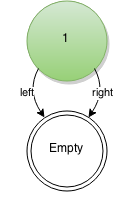`l.Add(2);``l = l.Add(2);`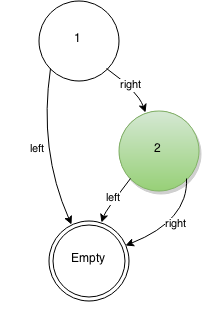`l.Add(3);``l = l.Add(3);`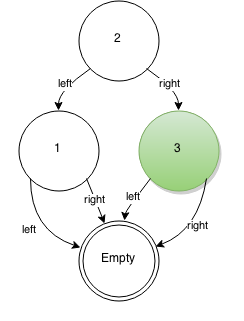`l.Add(4);`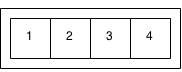`l = l.Add(4);`